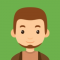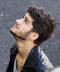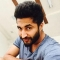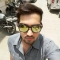## Difference between BFS and DFSUpdated on 20-Feb-2023 16:09:11
Both BFS and DFS are types of graph traversal algorithms, but they are different from each other. BFS or Breadth First Search starts from the top node in the graph and travels down until it reaches the root node. On the other hand, DFS or Depth First Search starts from the top node and follows a path to reaches the end node of the path. Read this article to learn more about these two graph traversal algorithms and how they are different from each other. What is BFS? Breadth First Search (BFS) algorithm traverses a graph in a breadth-ward motion ... Read More

## Bernoulli Distribution in Data StructuresUpdated on 27-Aug-2019 12:33:29
The Bernoulli Distribution is a discrete distribution having two possible outcomes labeled by x = 0 and x = 1. The x = 1 is success, and x = 0 is failure. Success occurs with probability p, and failure occurs with probability q as q = 1 – p. So$$P\lgroup x\rgroup=\begin{cases}1-p\:for & x = 0\p\:for & x = 0\end{cases}$$This can also be written as −$$P\lgroup x\rgroup=p^{n}\lgroup1-p\rgroup^{1-n}$$Example Live Demo#include #include using namespace std; int main(){    const int nrolls=10000;    default_random_engine generator;    bernoulli_distribution distribution(0.7);    int count=0; // count number of trues    for (int i=0; i

## Conversion of Gray Code to BinaryUpdated on 30-Jun-2020 11:15:22
The reflected binary code or Gray code is an ordering of the binary numeral system such that two successive values differ in only one bit (binary digit). Gray codes are very useful in the normal sequence of binary numbers generated by the hardware that may cause an error or ambiguity during the transition from one number to the next. So, the Gray code can eliminate this problem easily since only one bit changes its value during any transition between two numbers.Conversion of Gray to Binary CodeGray codes are used in rotary and optical encoders, Karnaugh maps, and error detection. The ... Read More

## What is Gray code?Updated on 30-Jun-2020 11:18:18
The reflected binary code or Gray code is an ordering of the binary numeral system such that two successive values differ in only one bit (binary digit). Gray codes are very useful in the normal sequence of binary numbers generated by the hardware that may cause an error or ambiguity during the transition from one number to the next. So, the Gray code can eliminate this problem easily since only one bit changes its value during any transition between two numbers.Gray code is not weighted that means it does not depends on positional value of digit. This cyclic variable code ... Read More

## What is Excess-3 Code?Updated on 30-Jun-2020 11:01:59
The excess-3 code (or XS3) is a non-weighted code used to express code used to express decimal numbers. It is a self-complementary binary coded decimal (BCD) code and numerical system which has biased representation. It is particularly significant for arithmetic operations as it overcomes shortcoming encountered while using 8421 BCD code to add two decimal digits whose sum exceeds 9. Excess-3 arithmetic uses different algorithm than normal non-biased BCD or binary positional number system.Representation of Excess-3 CodeExcess-3 codes are unweighted and can be obtained by adding 3 to each decimal digit then it can be represented by using 4 bit ... Read More

## Fixed Point and Floating Point Number RepresentationsUpdated on 30-Jun-2020 11:05:04
Digital Computers use Binary number system to represent all types of information inside the computers. Alphanumeric characters are represented using binary bits (i.e., 0 and 1). Digital representations are easier to design, storage is easy, accuracy and precision are greater.There are various types of number representation techniques for digital number representation, for example: Binary number system, octal number system, decimal number system, and hexadecimal number system etc. But Binary number system is most relevant and popular for representing numbers in digital computer system.Storing Real NumberThese are structures as following below −There are two major approaches to store real numbers (i.e., ... Read More

## 1's Complement vs 2's ComplementUpdated on 30-Jun-2020 11:05:57
Complements are used in digital computers in order to simply the subtraction operation and for the logical manipulations. For the Binary number (base-2) system, there are two types of complements: 1’s complement and 2’s complement.1’s Complement of a Binary NumberThere is a simple algorithm to convert a binary number into 1’s complement. To get 1’s complement of a binary number, simply invert the given number.2’s Complement of a Binary NumberThere is a simple algorithm to convert a binary number into 2’s complement. To get 2’s complement of a binary number, simply invert the given number and add 1 to the ... Read More

## Two’s ComplementUpdated on 02-Dec-2021 12:44:21
Binary Number System is one the type of most popular Number Representation techniques that used in digital systems. In the Binary System, there are only two symbols or possible digit values, i.e., 0 (off) and 1 (on). Represented by any device that only 2 operating states or possible conditions.Generally, there are two types of complement of Binary number: 1’s complement and 2’s complement. To get 1’s complement of a binary number, simply invert the given number. For example, 1’s complement of binary number 110010 is 001101. To get 2’s complement of binary number is 1’s complement of given number plus ... Read More

## One’s ComplementUpdated on 25-Jul-2020 12:59:11
Binary Number System is one the type of most popular Number Representation techniques that used in digital systems. In the Binary System, there are only two symbols or possible digit values, i.e., 0 (off) and 1 (on). Represented by any device that only 2 operating states or possible conditions.Generally, there are two types of complement of Binary number: 1’s complement and 2’s complement. To get 1’s complement of a binary number, simply invert the given number. For example, 1’s complement of binary number 110010 is 001101. To get 2’s complement of binary number is 1’s complement of given number plus ... Read More

## Negative Binary NumbersUpdated on 30-Jul-2019 22:30:24
Negative numbers can be distinguishable with the help of extra bit or flag called sign bit or sign flag in Binary number representation system for signed numbers. It is not possible to add minus or plus symbol in front of a binary number because a binary number can have only two symbol either 0 or 1 for each position or bit. That’s why we use this extra bit called sign bit or sign flag. The value of sign bit is 1 for negative binary numbers and 0 for positive numbers.When an integer binary number is positive, the sign is represented ... Read More